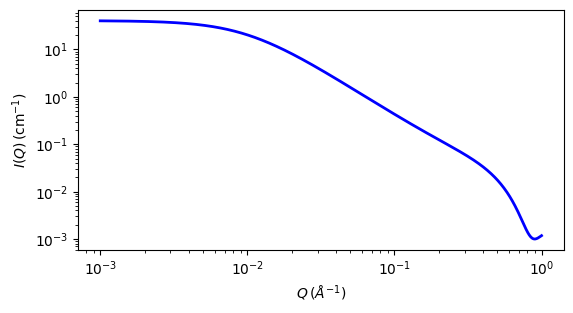# fractal

Calculates the scattering from fractal-like aggregates of spheres following theTexiera reference.

Parameter

Description

Units

Default value

scale

Scale factor or Volume fraction

None

1

background

Source background

cm-1

0.001

volfraction

volume fraction of blocks

None

0.05

5

fractal_dim

fractal dimension

None

2

cor_length

cluster correlation length

100

sld_block

scattering length density of particles

10-6-2

2

sld_solvent

scattering length density of solvent

10-6-2

6.4

The returned value is scaled to units of cm-1 sr-1, absolute scale.

Definition This model calculates the scattering from fractal-like aggregates of spherical building blocks according the following equation:

$I(q) = \phi\ V_\text{block} (\rho_\text{block} - \rho_\text{solvent})^2 P(q)S(q) + \text{background}$

where $$\phi$$ is The volume fraction of the spherical “building block” particles of radius $$R_0$$, $$V_{block}$$ is the volume of a single building block, $$\rho_{solvent}$$ is the scattering length density of the solvent, and $$\rho_{block}$$ is the scattering length density of the building blocks, and P(q), S(q) are the scattering from randomly distributed spherical particles (the building blocks) and the interference from such building blocks organized in a fractal-like clusters. P(q) and S(q) are calculated as:

$\begin{split}P(q)&= F(qR_0)^2 \\ F(q)&= \frac{3 (\sin x - x \cos x)}{x^3} \\ V_\text{particle} &= \frac{4}{3}\ \pi R_0 \\ S(q) &= 1 + \frac{D_f\ \Gamma\!(D_f-1)}{[1+1/(q \xi)^2\ ]^{(D_f -1)/2}} \frac{\sin[(D_f-1) \tan^{-1}(q \xi) ]}{(q R_0)^{D_f}}\end{split}$

where $$\xi$$ is the correlation length representing the cluster size and $$D_f$$ is the fractal dimension, representing the self similarity of the structure. Note that S(q) here goes negative if $$D_f$$ is too large, and the Gamma function diverges at $$D_f=0$$ and $$D_f=1$$.

Polydispersity on the radius is provided for.

For 2D data: The 2D scattering intensity is calculated in the same way as 1D, where the q vector is defined as

$q = \sqrt{q_x^2 + q_y^2}$Fig. 101 1D plot corresponding to the default parameters of the model.

Source

fractal.py $$\ \star\$$ fractal.c $$\ \star\$$ fractal_sq.c $$\ \star\$$ sas_gamma.c $$\ \star\$$ sas_3j1x_x.c

References

1. J Teixeira, J. Appl. Cryst., 21 (1988) 781-785

Authorship and Verification

• Author: NIST IGOR/DANSE Date: pre 2010

• Converted to sasmodels by: Paul Butler Date: March 19, 2016